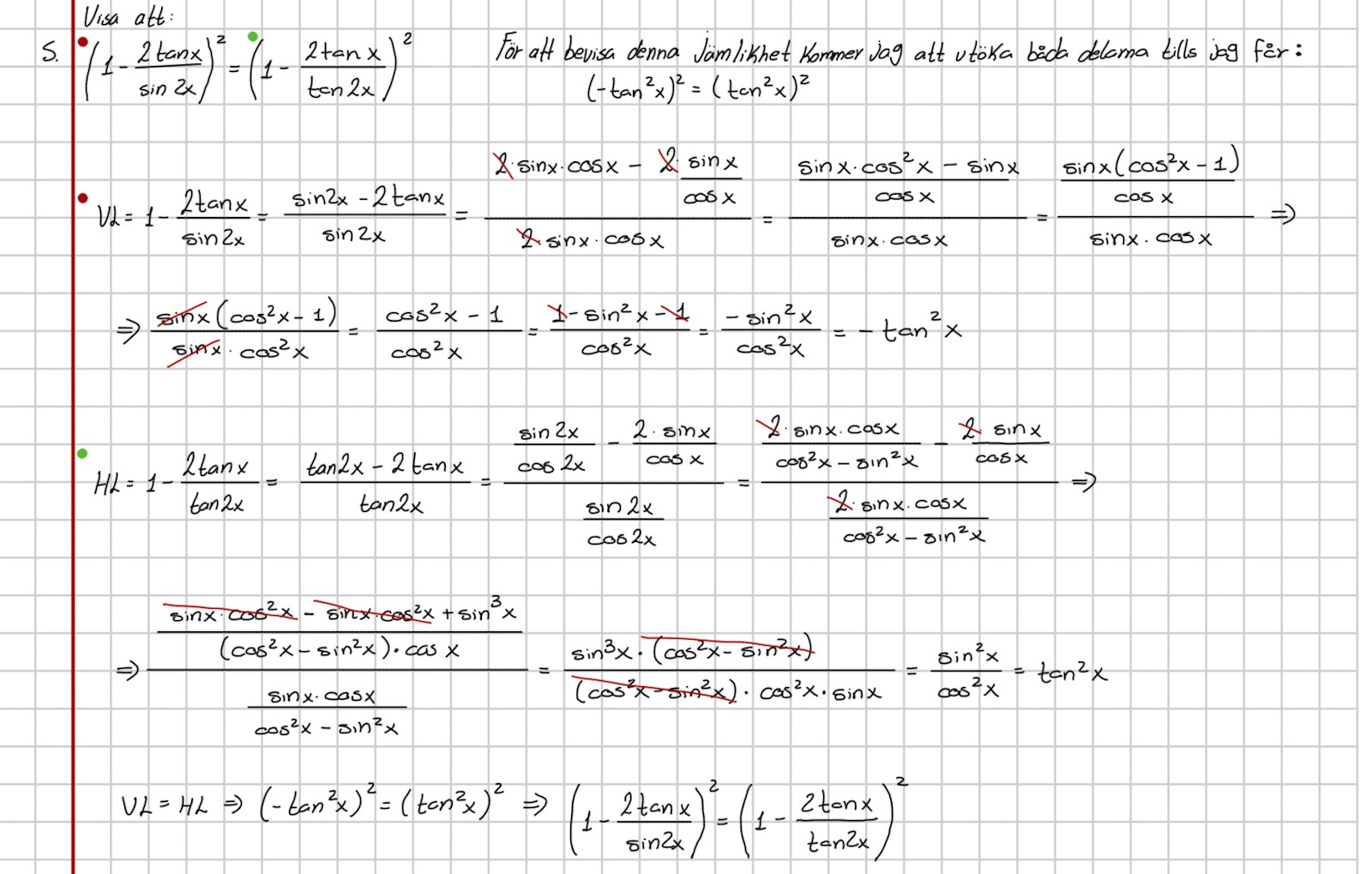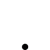7 svar
82 visningar
Lucho2502 är nöjd med hjälpen

# Bevisa denna jämlikhet.

I don't speak too much Swedish, so I hope you can help me in English or I can always use the translator. I couldn't find another way to prove it than the one I used. We have not study the double angle of the tangent so I guessed I had to use what I knew.

(sin(2x) = 2*sin(x)*cos(x))

(cos(2x)=cos^2(x)-sin^2(x))

(tan(2x)= sin(2x)/cos(2x))

I'd be really grateful if someone can say me if it's right my reasoning or if there's a simpler way.Put x=45 (degrees). Then sin 2x=sin 90=1, -2 tan x=-2*1=-2, so left side =1-2=-1. But tan 2x tends to infinity when  x tends to 45 so tan 2x is not defined for this angle. Therefore, even if the singularity in the right side is nicely removable, the equality does not hold everywhere. When the singularity  is removed the equality is another than the the given one. What about x=0?

At a quick glance on your calculations I can not find any flaws.

Det heter förresten ekvation, inte jämlikhet.

Inequality heter däremot olikhet.

Tomten skrev:

Put x=45 (degrees). Then sin 2x=sin 90=1, -2 tan x=-2*1=-2, so left side =1-2=-1. But tan 2x tends to infinity when  x tends to 45 so tan 2x is not defined for this angle. Therefore, even if the singularity in the right side is nicely removable, the equality does not hold everywhere. When the singularity  is removed the equality is another than the the given one. What about x=0?

At a quick glance on your calculations I can not find any flaws.

Thanks for the help.

The thing is that we should be able to prove the equality without substituting the angles. Like if I had tan(x)=sin(x)/cos(x)

To prove this one I just need to know the fundamental identity of tan(x) which means that I know that tan(x) can be replaced by sin(x)/cos(x) so both sides of the equality are the same.

Laguna skrev:

Det heter förresten ekvation, inte jämlikhet.

Inequality heter däremot olikhet.

Thanks for the help. I'm sorry but in this case it's a "jämlikhet".

I'm not trying to solve an equation. The exercise is about to demonstrate that knowing the trigonometric identities you can prove that one side of the equality is equal to the other by changing for example tan(x) for sin(x)/cos(x) and so on.